#### 相似文章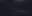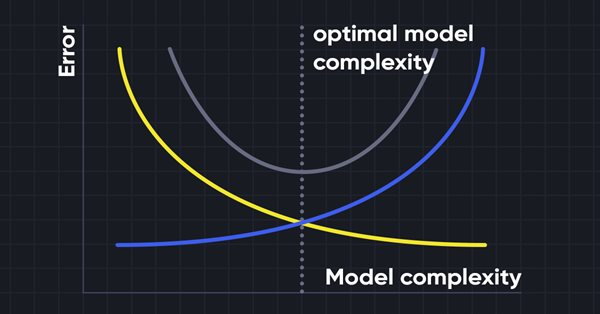# 数据科学与机器学习（第 10 部分）：岭回归

MetaTrader 5统计分析 | 2 五月 2023, 11:009550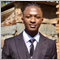### 概述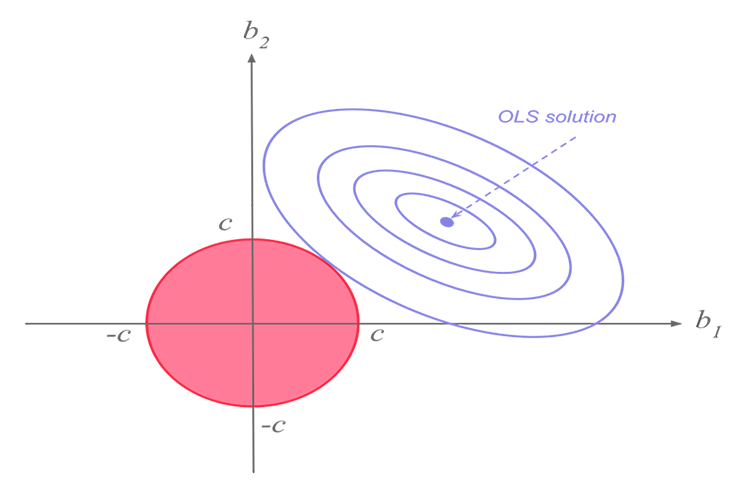### 这对模型意味着什么？

• 低偏差：具有低偏差的模型对目标函数的形式做出较少的假设
• 高偏差：具有高偏差的模型做出更多假设，并且能够捕获训练数据集内的关系

### 方差

方差告知随机变量距其预期值的差异程度。
减少高偏差的途径。
• 在模型拟合不足时增加输入特征
• 减少正则化项
• 采用更复杂的特征，例如包括一些多项式特征

减少高方差的途径。

• 减少输入特征；在模型拟合不足时的参数数量
• 不要采用太复杂的模型
• 增加训练数据
• 增加正则化项

### 偏差-方差权衡

在构建机器学习模型时，注意偏差和方差从而避免模型过度拟合非常重要。 如果模型非常简单，且参数较少，则它往往具有较大的偏差，但方差较小；而复杂模型通常最终具有低偏差，但高方差值。 故此需要在偏差和方差误差之间取得平衡，在这两项之间找到平衡称为偏差-方差权衡。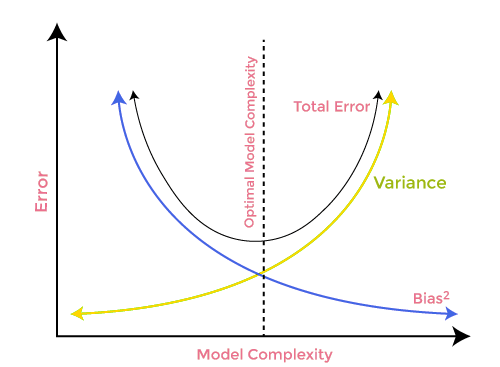为了准确预测模型，算法也需要较低的偏差和较低的方差，但这实际上是不可能的，因为偏差和方差彼此呈负相关。
如果我们增加偏差，方差就会减小，反之亦然。

### 岭回归

岭回归和 lasso 回归两者都处于相同任务上，但它们有一个重大差别，我们将在稍后深入研究数学理论、并尝试搞清楚是什么让每个算法起作用时看到这一点。

### 岭回归背后的思路。

当我们有很多线性相关的测量值时，我们可以确信最小二乘法可以很好地反映自变量和目标变量之间的关系。

看看下面的老鼠的大小与其体重的关系图示例。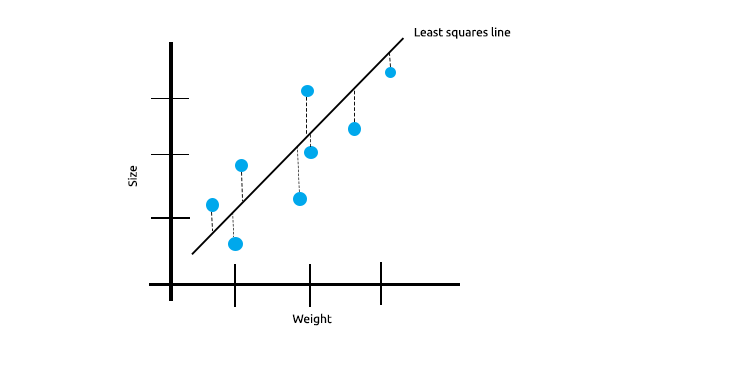但是，如果只取两个测量值作为我们的训练数据集，其余的作为我们的测试数据集，那么用最小二乘拟合模型将产生完美拟合，从而得到残差平方零和。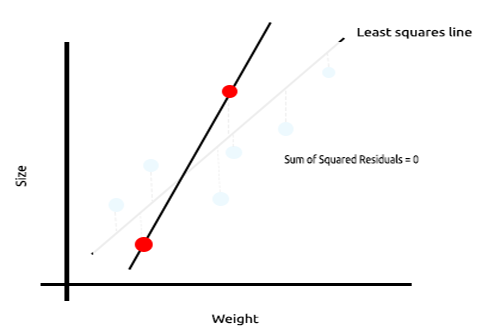现在我们在新数据集上测试此模型；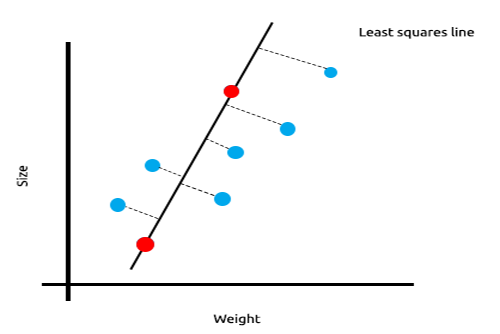训练数据的平方误差总和为零，但测试数据的残差平方和很大，这意味着我们的模型具有很高的方差，在机器学习术语中，我们说该模型与训练数据过度拟合。

岭回归和 lasso 回归背后的主要思想是找到不适合训练数据的模型。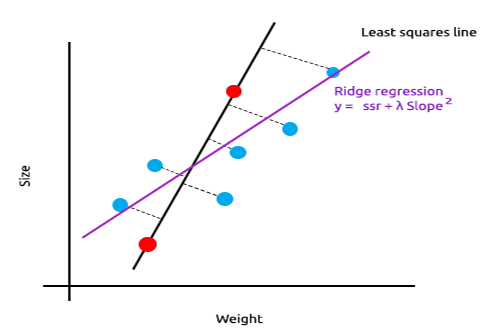在岭回归中，通过将少量偏差引入新线，我们得到明显的方差降低。 由于岭回归引入了少量偏差，因此模型现在不能很好地拟合训练数据和测试数据，但从长远来看，它却为我们提供了一个可靠的模型。

### 何时能使用这些正则化模型。

有人可能会问自己，如果最小二乘法/线性回归模型就可以很好地完成，为什么要使用这个 L1norm 和 L2norm 模型？

为了理解这一点，我们来看看多变量线性回归在训练数据集上的表现。

为了更好地说明我试图表达的观点，我针对 EURUSD 准备了振荡指标和交易量指标的全部数据集；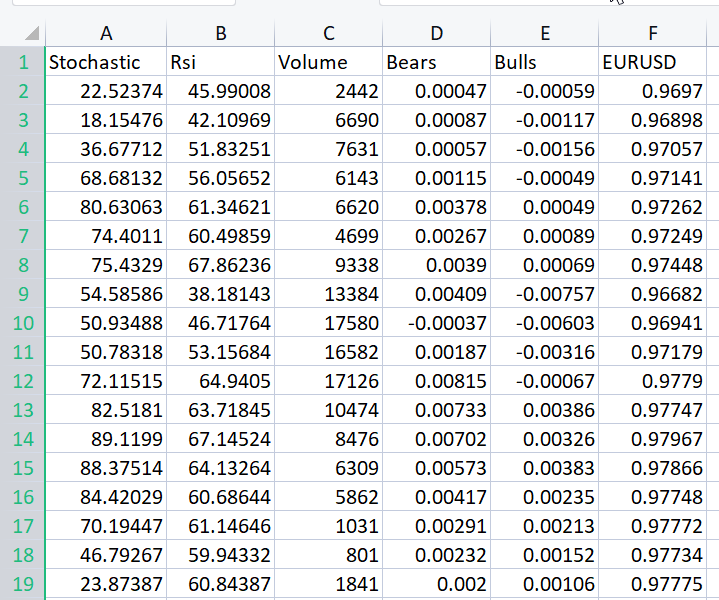甚至不用看相关矩阵，每个熟悉这些指标的人都肯定知道这些指标不适合回归问题。 下面是相关矩阵

```    ArrayPrint(matrix_utils.csv_header);
Print(Matrix.CorrCoef(false));```

结果:

```CS      0       06:29:41.493    TestEA (EURUSD,H1)      "Stochastic" "Rsi"        "Volume"     "Bears"      "Bulls"      "EURUSD"
CS      0       06:29:41.493    TestEA (EURUSD,H1)      [[1,0.680705511991766,0.02399740959375265,0.6910892641498844,0.7291018045506749,0.1490856367010467]
CS      0       06:29:41.493    TestEA (EURUSD,H1)       [0.680705511991766,1,0.07620207894739518,0.8184961346648213,0.8258569040865805,0.1567269000583347]
CS      0       06:29:41.493    TestEA (EURUSD,H1)       [0.02399740959375265,0.07620207894739518,1,0.3752014290536041,-0.1289026185114097,-0.1024017077869821]
CS      0       06:29:41.493    TestEA (EURUSD,H1)       [0.6910892641498844,0.8184961346648213,0.3752014290536041,1,0.7826404088603456,0.07283638913665436]
CS      0       06:29:41.493    TestEA (EURUSD,H1)       [0.7291018045506749,0.8258569040865805,-0.1289026185114097,0.7826404088603456,1,0.08392530400705019]
CS      0       06:29:41.493    TestEA (EURUSD,H1)       [0.1490856367010467,0.1567269000583347,-0.1024017077869821,0.07283638913665436,0.08392530400705019,1]]```
如您所见，EURUSD 列与所有指标的相关性低于 20%，随机指标和 RSI 似乎比其它指标似乎具有最佳相关性，但也分别只是约 14%15%。 我们创建一个线性回归模型，从随机指标开始，然后我们将继续添加自变量/其它指标读数。

结果表格:

自变量 R2 得分(精确度)
随机振荡器     1.2 %
随机振荡器和 RSI     1.8 %
随机振荡器, RSI 和交易量     2.8 %
随机振荡器, RSI, 交易量, 看跌推力, 和看涨推力   (所有自变量)     4.9%

那么，您能从这张表格里得出什么结论呢？随着自变量数量的增加，无论这些变量是什么，训练好的线性模型的准确度总是会增加；我在这个例子中所用的自变量的相关性非常低，这就是为什么每次添加新的自变量时，您会看到准确度都只略有提高；但当变量相关度大约 30%40% 时，情况可能并非如此，当您为提供太多这些自变量时，您也许能见证模型在训练阶段达到 90% 的准确率。

自变量的增加同时导致方差增加，毫无疑问，该模型在新数据集中的表现会更差，因为它是过度拟合的；为了解决这个问题，引入了岭回归和 lasso 回归，如前所述，通过添加某种偏差，我们得到了明显的方差降低。

### 岭回归理论

岭回归本身是一种在自变量高度相关时估侧线性回归模型系数的方法。

开发岭回归是当线性回归模型具有一些多重共线性（高度相关）时，针对最小二乘估计器不精确性的可能解决方案 — 通过创建岭回归估计器（RR）。 这提供了更精确的岭参数，因为它的方差和偏差通常小于最小二乘估计量。

### 岭回归估测器

类似于普通的最小二乘估测器，简单的岭估计器由下式给出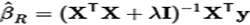其中 y 是自变量矩阵，X 是设计矩阵，I 是单位矩阵，而岭参数 λ 是大于或等于零的数值。

我们为此编写代码：

```CRidgeregression::CRidgeregression(matrix &_matrix)
{

n = _matrix.Rows();
k = _matrix.Cols();

pre_processing.Standardization(_matrix);
m_dataset.Copy(_matrix);

matrix_utils.XandYSplitMatrices(_matrix,XMatrix,yVector);

YMatrix = matrix_utils.VectorToMatrix(yVector);

//---

Id_matrix.Resize(k,k);

Id_matrix.Identity();

}
```

在函数构造函数上，有三件重要的事情要完成，首先是标准化数据。 就像多变量梯度下降和许多其它机器学习技术一样，岭回归在标准化数据集中工作，其次将数据分成 x 和 y 矩阵，最后创建恒等矩阵。

在 L2Norm 函数内部:

```vector CRidgeregression::L2Norm(double lambda)
{
matrix design = matrix_utils.DesignMatrix(XMatrix);

matrix XT = design.Transpose();

matrix XTX = XT.MatMul(design);

matrix lamdaxI = lambda * Id_matrix;

//Print("LambdaxI \n",lamdaxI);

//Print("XTX\n",XTX);

matrix sum_matrix = XTX + lamdaxI;

matrix Inverse_sum = sum_matrix.Inv();

matrix XTy = XT.MatMul(YMatrix);

Betas = Inverse_sum.MatMul(XTy);

#ifdef DEBUG_MODE
Print("Betas\n",Betas);
#endif

return(matrix_utils.MatrixToVector(Betas));
} ```

这个函数遵照我们刚刚看到的上述公式的指导执行所有操作，并运用岭回归查找系数。.

为了看明白其工作原理，我们用本系列文章读者已经熟悉的另一个数据集 NASDAQ_DATA.csv。

```int OnInit()
{
//---

pre_processing.Standardization(Matrix);
Linear_reg = new  CLinearRegression(Matrix);

ridge_reg = new CRidgeregression(Matrix);

ridge_reg.L2Norm(0.3);
}    ```

我为岭回归设置了 0.3 的随机惩罚值，如此我们就能看到由此产生的结果。 现在，是时候运行函数，并查看由此得到的系数了；

CS 0 10:27:41.338 TestEA (EURUSD,H1) [[5.015577002384403e-16]

CS 0 10:27:41.338 TestEA (EURUSD,H1) [0.6013523727380532]

CS 0 10:27:41.338 TestEA (EURUSD,H1) [0.3381524618200134]

CS 0 10:27:41.338 TestEA (EURUSD,H1) [0.2119467984461254]]

我们针对同一数据集也运行线性回归模型，并观察它们的系数；由于最小二乘法不会标准化数据集，因此在将数据提供给模型之前，我们先对其进行标准化。

```    matrix Matrix = matrix_utils.ReadCsv("NASDAQ_DATA.csv",",");

pre_processing.Standardization(Matrix);
Linear_reg = new  CLinearRegression(Matrix);```

输出:

CS 0 10:27:41.338 TestEA (EURUSD,H1) Betas

CS 0 10:27:41.338 TestEA (EURUSD,H1) [[-4.143037461930866e-14]

CS 0 10:27:41.338 TestEA (EURUSD,H1) [0.6034777119810752]

CS 0 10:27:41.338 TestEA (EURUSD,H1) [0.3363532376334173]

CS 0 10:27:41.338 TestEA (EURUSD,H1) [0.21126507562567]]

系数看起来只是略有不同，故我猜我们的函数有效。我们来训练和测试每个模型，然后最终绘制它们各自的图形，以便了解更多信息。

由于岭回归本身不是一个模型，而是需要与线性回归模型一起使用的系数的估测器，因此我对在第 3 部分中讨论的线性回归类进行了一些更改。

在线性回归中，类构造函数是模型进行训练的所在。 这个区域当中存储的系数，之后供其余函数所用，我已添加了一个新的构造函数，允许将系数传递给模型，这将有助于我们在下次使用其它估测器时以最小的工作量来获取我们希望回归模型采用的系数。

```class CLinearRegression
{
public:
CLinearRegression(matrix &Matrix_); //Least squares estimator
CLinearRegression(matrix<double> &Matrix_, double Lr, uint iters = 1000); //Lr by Gradient descent
CLinearRegression(matrix &Matrix_, vector &coeff_vector);

~CLinearRegression(void);```

### 岭回归与线性回归

```    Print("----> Ridge regression");

ridge_reg = new CRidgeregression(Matrix);
vector coeff = ridge_reg.L2Norm(0.3);

Linear_reg = new CLinearRegression(Matrix,coeff); //passing the coefficients made by ridge regression
// to the Linear regression model
double acc =0;

vector ridge_predictions = Linear_reg.LRModelPred(Matrix,acc); //making the predictions and storing them to a vector

delete(Linear_reg); //deleting that instance

Print("----> Linear Regression");

pre_processing.Standardization(Matrix);

Linear_reg = new CLinearRegression(Matrix); //new Linear reg instance that gets coefficients by least squares

vector linear_pred = Linear_reg.LRModelPred(Matrix,acc); ```

输出:

CS 0 11:35:52.153 TestEA (EURUSD,H1) ----> Ridge regression

CS 0 11:35:52.153 TestEA (EURUSD,H1) Betas

CS 0 11:35:52.153 TestEA (EURUSD,H1) [[-4.142058558619502e-14]

CS 0 11:35:52.153 TestEA (EURUSD,H1) [0.601352372738047]

CS 0 11:35:52.153 TestEA (EURUSD,H1) [0.3381524618200102]

CS 0 11:35:52.153 TestEA (EURUSD,H1) [0.2119467984461223]]

CS 0 11:35:52.154 TestEA (EURUSD,H1) R squared 0.982949 Adjusted R 0.982926

CS 0 11:35:52.154 TestEA (EURUSD,H1) ----> Linear Regression

CS 0 11:35:52.154 TestEA (EURUSD,H1) Betas

CS 0 11:35:52.154 TestEA (EURUSD,H1) [[5.014846059117108e-16]

CS 0 11:35:52.154 TestEA (EURUSD,H1) [0.6034777119810601]

CS 0 11:35:52.154 TestEA (EURUSD,H1) [0.3363532376334217]

CS 0 11:35:52.154 TestEA (EURUSD,H1) [0.2112650756256718]]

CS 0 11:35:52.154 TestEA (EURUSD,H1) R squared 0.982933 Adjusted R 0.982910

当您将所有数据用作训练数据时，模型的性能略有不同。

当存储输出，并在同一轴上绘制时，这是它们的图形;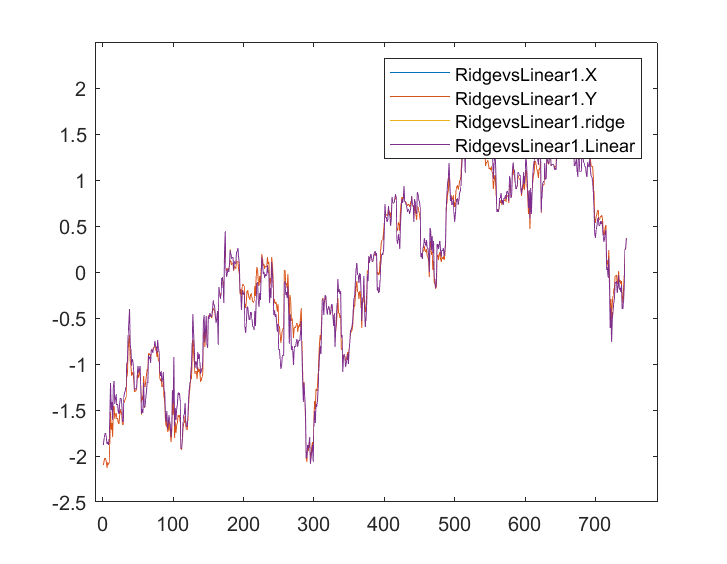我几乎看不出线性模型与蓝色标记的预测变量之间的任何差别，我只能看到两个模型之间的差异，并且岭回归不太适合该数据集，这是个好消息。 我们来逐个训练和测试这两个模型。

```    matrix_utils.TrainTestSplitMatrices(Matrix,TrainMatrix,TestMatrix);

Print("----> Ridge regression | Train ");

ridge_reg = new CRidgeregression(TrainMatrix);
vector coeff = ridge_reg.L2Norm(0.3);

Linear_reg = new CLinearRegression(TrainMatrix,coeff); //passing the coefficients made by ridge regression
// to the Linear regression model
Linear_reg.LRModelPred(TrainMatrix,acc);

printf("Accuracy %.5f ",acc);

Print("----> Ridge regression | Test");

vector ridge_predictions = Linear_reg.LRModelPred(TestMatrix,acc); //making the predictions and storing them to a vector

printf("Accuracy %.5f ",acc);

delete(Linear_reg); //deleting that instance

Print("\n----> Linear Regression | Train ");

Linear_reg = new CLinearRegression(TrainMatrix); //new Linear reg instance that gets coefficients by least squares

Linear_reg.LRModelPred(TrainMatrix,acc);

printf("Accuracy %.5f ",acc);

Print("----> Linear Regression | Test ");

vector linear_pred = Linear_reg.LRModelPred(TestMatrix,acc);

printf("Accuracy %.5f ",acc);
```

输出:

CS 0 13:27:40.744 TestEA (EURUSD,H1) ----> Ridge regression | Train

CS 0 13:27:40.744 TestEA (EURUSD,H1) Accuracy 0.97580

CS 0 13:27:40.744 TestEA (EURUSD,H1) ----> Ridge regression | Test

CS 0 13:27:40.744 TestEA (EURUSD,H1) Accuracy 0.78620

CS 0 13:27:40.744 TestEA (EURUSD,H1)

CS 0 13:27:40.744 TestEA (EURUSD,H1) ----> Linear Regression | Train

CS 0 13:27:40.744 TestEA (EURUSD,H1) Accuracy 0.97580

CS 0 13:27:40.744 TestEA (EURUSD,H1) ----> Linear Regression | Test

CS 0 13:27:40.744 TestEA (EURUSD,H1) Accuracy 0.78540

看起来两个模型在训练中的准确度大致相同，但在测试数据集中略有不同，考虑到岭回归所采用的惩罚自变量 0.3 很小，这其实还不错；我们还不清楚如何选择正确的惩罚。

当我将 lambda 值设置为 10 时，岭回归训练精度从 0.97580 下降到 0.95760，而测试精度从 0.78540 上升到 0.80050当然只是略有增长

### 选择正确的惩罚值（lambda）

为了寻找正确的 lambda 值，我们需要利用留一交叉验证法（LEAVE ONE OUT CROSS VALIDATION — LOOCV）技术，对于那些不熟悉它的人来说，这是在 ML 中找到某些模型的最佳参数的技术；它实现的方式是遍历所有数据集，从数据集中保留一个样本，然后用数据集的其余部分训练模型，即 n-1；然后再用保留的那个数据作为测试样本，它遍历所有数据集，直到第 n 个样本，最后测量每次迭代中所有值的损失，最后它找到 lambda 值的最小损失函数的所在，产生误差最小的函数是最佳参数，以备读取更多信息。

我们导入交叉验证类来帮助我们找到 lambda 的最佳值。

```#include <MALE5\cross_validation.mqh>
CCrossValidation *cross_validation;```

以下是岭回归的 LOOCV 代码；

```double CCrossValidation::LeaveOneOut(double init, double step, double finale)
{
matrix XMatrix;
vector yVector;

matrix_utils.XandYSplitMatrices(Matrix,XMatrix,yVector);

matrix train = Matrix; vector test = {};

int size = int(finale/step);
vector validation_output(ulong(size));
vector lambda_vector(ulong(size));

vector forecast(n);
vector actual = yVector;

double lambda = init;

for (int i=0; i<size; i++)
{
lambda += step;

for (ulong j=0; j<n; j++)
{
train.Copy(Matrix);
ZeroMemory(test);

test = XMatrix.Row(j);

matrix_utils.MatrixRemoveRow(train,j);

vector coeff = {};
double acc =0;

switch(selected_model)
{
case  RIDGE_REGRESSION:

ridge_regression = new CRidgeregression(train);
coeff = ridge_regression.L2Norm(lambda); //ridge regression

Linear_reg = new CLinearRegression(train,coeff);

forecast[j] =  Linear_reg.LRModelPred(test);

//---

delete (Linear_reg);
delete (ridge_regression);

break;
}
}

validation_output[i] = forecast.Loss(actual,LOSS_MSE)/double(n);

lambda_vector[i] = lambda;

#ifdef DEBUG_MODE
printf("%.5f LOOCV mse %.5f",lambda_vector[i],validation_output[i]);
#endif
}

//---

#ifdef  DEBUG_MODE
matrix store_matrix(size,2);

store_matrix.Col(validation_output,0);
store_matrix.Col(lambda_vector,1);

string name = EnumToString(selected_model)+"\\LOOCV.csv";

#endif

return(lambda_vector[validation_output.ArgMin()]);
}
```

我们将其付诸行动；

```int OnInit()
{

ridge_reg = new CRidgeregression(Matrix);

cross_validation = new CCrossValidation(Matrix,RIDGE_REGRESSION);

double best_lambda = cross_validation.LeaveOneOut(0,1,10);

Print("Best lambda ",best_lambda);```

输出:

```CS      0       10:12:51.346    ridge_test (EURUSD,H1)  1.00000 LOOCV mse 0.00020
CS      0       10:12:51.465    ridge_test (EURUSD,H1)  2.00000 LOOCV mse 0.00020
CS      0       10:12:51.576    ridge_test (EURUSD,H1)  3.00000 LOOCV mse 0.00020
CS      0       10:12:51.684    ridge_test (EURUSD,H1)  4.00000 LOOCV mse 0.00020
CS      0       10:12:51.788    ridge_test (EURUSD,H1)  5.00000 LOOCV mse 0.00020
CS      0       10:12:51.888    ridge_test (EURUSD,H1)  6.00000 LOOCV mse 0.00020
CS      0       10:12:51.987    ridge_test (EURUSD,H1)  7.00000 LOOCV mse 0.00021
CS      0       10:12:52.090    ridge_test (EURUSD,H1)  8.00000 LOOCV mse 0.00021
CS      0       10:12:52.201    ridge_test (EURUSD,H1)  9.00000 LOOCV mse 0.00021
CS      0       10:12:52.317    ridge_test (EURUSD,H1)  10.00000 LOOCV mse 0.00021
CS      0       10:12:52.319    ridge_test (EURUSD,H1)  Best lambda 1.0```

假设代码中没有漏洞，则 lambda 的最佳值是搜索从 1 到 10 时的值。 这告诉我们该模型的 lambda 值略小，故此我决定从 0 到 10 循环，步长设置为 0.01（总共 1000 次迭代），这需要花费 5 分钟才能完成，但是，我能得到 0.09 的值作为 lambda 的最佳值， 下面是其绘图;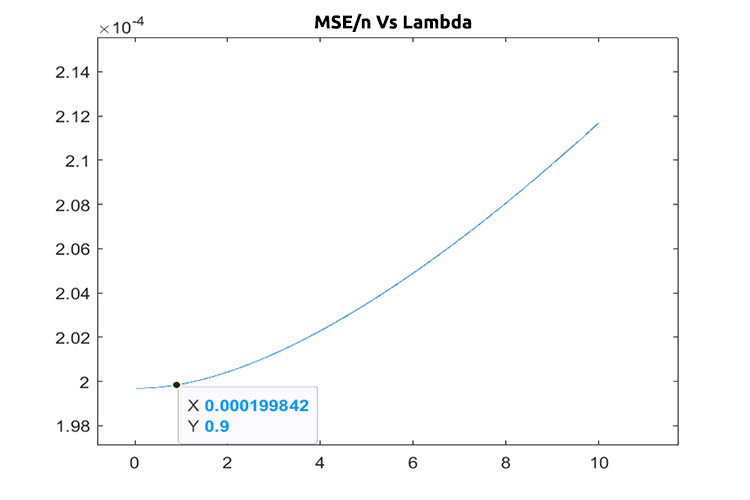酷！现在岭回归的部分一切都很好。

### 岭回归的优点

• 我们看看运用岭回归估测器的一些益处
•  它可以防止模型过度拟合
•  降低模型复杂度
•  它对于多变量数据集中的线性回归表现良好
•  不需要无偏估测器

### 岭回归的缺点

•  它包括最终模型中的所有预测变量
•  它无法执行特征选择
•  它将系数缩小到零
•  它用方差换取偏差

### 后记

在存在多变量的情况下，岭回归可能有助于避免回归模型过度拟合，但需您从模型中手工避免/删除不需要的变量，这仍然至关重要，从我们的 NASDAQ_DATA，我们本可以删除 RSI 列，因为我们所有人都可能知道它与我们的目标变量无关。对于本文的内容，我暂时无法涵盖的内容太多了。

请持续跟踪我在 GitHub 存储库上的岭回归开发进度 > https://github.com/MegaJoctan/MALE5

文件名 说明
cross_validation.mqh  如同 sklearn 交叉验证一样，此文件包含验证技术，如 LOOCV
Linear regression.mqh  此文件包含最小二乘法/ 线性回归模型
matrix_utils.mqh  此实用工具类函数包含附加的矩阵运算函数
Preprocessing.mqh         如同 sklearn.preprocessing 一样，该类包含操作和重新缩放数据集的函数。
Ridge Regression.mqh  此文件包含岭回归模型及其相关函数
ridge_test.mq5  这是一个脚本，可测试我们在本文中讨论的所有内容
prepare_dataset.mq5  此脚本为我们之前讨论的振荡指标创建一个数据集。 这些数据将存储在一个文件 Oscillators.csv
NASDAQ_DATA.csv  此 csv 文件包含我们在本文中用到的数据集

本文由MetaQuotes Ltd译自英文
原文地址： https://www.mql5.com/en/articles/11735

附加的文件 |
MQL5.zip (142.77 KB)

#### 该作者的其他文章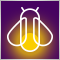种群优化算法：萤火虫算法（FA）
在本文中，我将研究萤火虫算法（FA）优化方法。 致谢优化修订，该算法已从局外人变成了评级表上的真正领先者。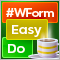DoEasy. 控件（第三十部分）：动画态滚动条控件
在本文中，我将继续开发滚动条（ScrollBar）控件，并开始实现鼠标交互功能。 此外，我将扩展鼠标状态标志和事件的列表。DoEasy. 控件（第三十一部分）：滚动条控件内内容的滚动
在本文中，我将实现通过按钮滚动水平滚动条容器内容的功能。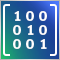矩阵实用工具，扩展矩阵和向量的标准库功能
矩阵作为机器学习算法和计算机的基础，因为它们能够有效地处理大型数学运算，标准库拥有所需的一切，但让我们看看如何在实用工具文件中引入若干个函数来扩展它，这些函数在标准库中尚未提供。